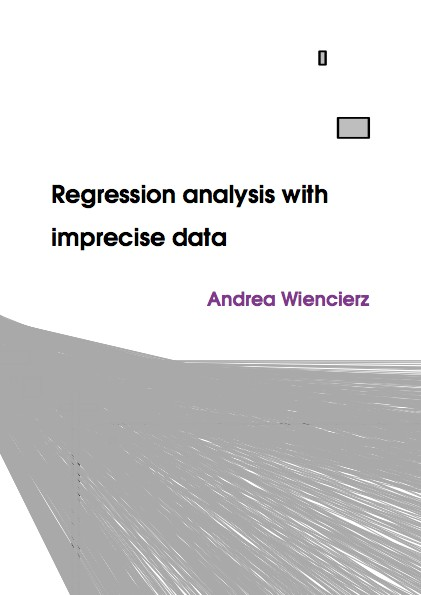Datenbestand vom 16. September 2021aktualisiert am 16. September 2021

# ISBN 978384391463572,00 € inkl. MwSt, zzgl. Versand

978-3-8439-1463-5, Reihe Statistik

Andrea Wiencierz
Regression analysis with imprecise data

137 Seiten, Dissertation Ludwig-Maximilians-Universität München (2013), Softcover, A5

## Zusammenfassung / Abstract

Statistical methods usually require that the analyzed data are correct and precise observations of the variables of interest. In practice, however, often only incomplete or uncertain information about the quantities of interest is available. The question studied in the present thesis is, how a regression analysis can reasonably be performed when the variables are only imprecisely observed.

At first, different approaches to analyzing imprecisely observed variables that were proposed in the Statistics literature are discussed. Then, a new likelihood-based methodology for regression analysis with imprecise data called Likelihood-based Imprecise Regression is introduced. The corresponding methodological framework is very broad and permits accounting for coarsening errors, in contrast to most alternative approaches to analyzing imprecise data. The methodology suggests considering as the result of a regression analysis the entire set of all regression functions that cannot be excluded in the light of the data, which can be interpreted as a confidence set. In the subsequent chapter, a very general regression method is derived from the likelihood-based methodology. This regression method does not impose restrictive assumptions about the form of the imprecise observations, about the underlying probability distribution, and about the shape of the relationship between the variables. Moreover, an exact algorithm is developed for the special case of simple linear regression with interval data and selected statistical properties of this regression method are studied. The proposed regression method turns out to be robust in terms of a high breakdown point and to provide very reliable insights in the sense of a set-valued result with a high coverage probability. In addition, an alternative approach proposed in the literature based on Support Vector Regression is studied in detail and generalized by embedding it into the framework of the formerly introduced likelihood-based methodology. In the end, the discussed regression methods are applied to two practical questions.